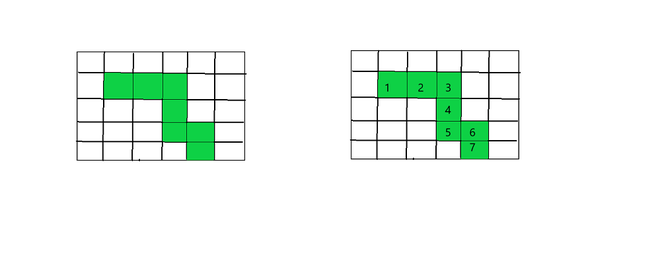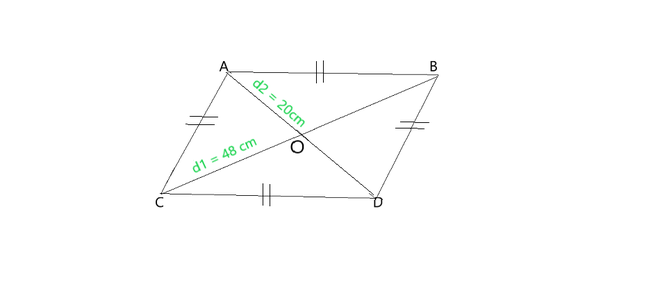Which figure encloses more area: a square of side 2cm or a rectangle of length 3cm and breadth 2cm?

• Last Updated : 09 Nov, 2021

Area is defined as the amount of space occupied by a 2-dimensional shape. In a different way, we can say that it is the quantity that calculates the number of unit squares that cover the surface of any closed/bounded figure. The standard unit of area is square units which are generally represented as square meter, square feet, square inch, square centimeter, etc.

The area of a closed shape is calculated with the help of its length and width. Length is unidirectional and its unit is meter (m), centimeter (cm), yards (yd), inches (in), etc. However, the area of a shape is a two-dimensional quantity i.e. the product of length and width. Hence, it is measured in square units like a square centimeter or (cm2), square meters (m2) square feet, or (ft 2), etc.

How To Measure Area Of Any Figure?

We will understand the concept with the help of grids, Each grid is made up of many squares of sides 1 unit by 1 unit. The area of each of these squares is 1 square unit. therefore we can say that each square is 1 square unit Look at the figure shown below. Let us find the area of the shape drawn in the grid.The area of the colored region is the number of shaded units squares

The area of the shape = 7 square units. because each box is one square unit, and there are 7 colored squares in total therefore, its square is 7 square units.

Area Of Different Geometric Shapes

if l is the length, b is breadth and h is the height of the geometric shape.

Area of Square

Area of square = side × side = side2 square units.

For example: if side of square is 6 m then its area will be 6 × 6 m2 = 36 m2

Area of Rectangle

Area of rectangle = length × breadth square units

for example: if the length and breadth of rectangle are 6 and 8 cm then its area will be 6 × 8 cm2 = 48 cm2

Area of Circle

Area of circle = π r2 square units

r is the radius of circle

for example: if radius of circle is 7 cm then its area is π 7 × 7 = 22/7 × 7 × 7 = 154 cm2

Area of triangle

Area of triangle = 1/2 × base × height = b × h square units

for example: if base and height of triangle are 4 and 6 cm respectively, then area of triangle is 1/2 × 4 × 6 = 12 cm2

Area of a parallelogram

Area of a parallelogram = base × height = b × h square units

for example if base and height of triangle are 5 and 6 cm respectively, then area of parallelogram is 5 × 6 = 30 cm2.

Area of Rhombus

Area of Rhombus = 1/2 × (d1) × (d2) square units

Which figure encloses more area: a square of side 2cm or a rectangle of length 3cm and breadth 2cm?

Solution:

First consider, square

Length of square = 2 cm

Area of square = side2

A = 22

A = 4 cm2

Now, consider rectangle

length of rectangle = 3 cm

breadth of rectangle = 2 cm

Area of rectangle = length × breadth = 3 × 2 cm2

A’ = 6 cm2

Now after comparing values of A and A’ we see that the area of A’ is greater which means that area of rectangle is greater than square.

Sample Problems

Question 1: The side of a square is 7 cm, calculate its Area.

Solution:

Side of square = 7 cm

Area of a square = side × side.

A = 7 × 7 = 49

Therefore, the area of the square = 49 cm2.

Question 2: If the area of a rectangle is 120 square cm and one of its dimensions is 15cm calculate the other.

Solution:

Given,

let length of rectangle, l = 15 cm

Area of rectangle, A = 120 cm2.

Area of rectangle = length × breadth

120 = 15 × b

=>       b = 120/15

b = 8 cm.

Hence, other dimension of rectangle is 8cm.

Question 3: What will be the length of the rectangle, if the area of a rectangle of width 5 cm, is equal to the area of a square of side 10 cm?

Solution:

Area of square = side x side

A = 10 × 10 square cm.

A = 100 square cm

Now,

Area of rectangle = length × breadth

A’ = l × 5

A’ = 5l square cm

According to question :

Area of square = Area of rectangle

=>     A’ = A

5l = 100

l = 100/5 = 20 cm

Length of rectangle is 20cm

Question 4: If the diagonals of a rhombus are 20 and 48 cm, Find the perimeter of the rhombus.

Solution:

Given diagonal d1 = 48 cm

diagonal d2 = 20 cmRhombus with diagonals 48 and 20 cm

OA = AD/2 = 20/2 = 10cm

OC = BC/2 = 48/2 = 24cm

In triangle AOC

Using pythagoras theorem

AC2 = OA2+ OC2

AC = √(10)2 + (24)2

AC = √100+ 576 = √676 = 26cm

Perimeter of rhombus = 4 × side

= 4 × 26 = 104cm

Question 5: What will happen to the area of the square if its side becomes double.

Solution:

Let, the side of square be s

now the Area of square = side × side

A = s2 square cm

If the side s doubles then, New side

s’ = 2s

Area of new square = side × side= s’ × s’

A’ = 2s × 2s  = 4s2

A’ = 4 × A  —— (A = s2

=> Area of square will increases four times if we double its side.

Question 6: What will happen to the area of the square if its side becomes half.

Solution:

Let, the side of square be s

Now the Area of square = side × side

A = s2 square cm

If the side s half then, New side

s’ = s/2

Area of new square = side × side = s’ × s’

A’ = s/2 × s/2 = s2/4

A’ = A/4  —— (A = s2)

=> Area of square will decrease to one-forth times if we half its side.

My Personal Notes arrow_drop_up